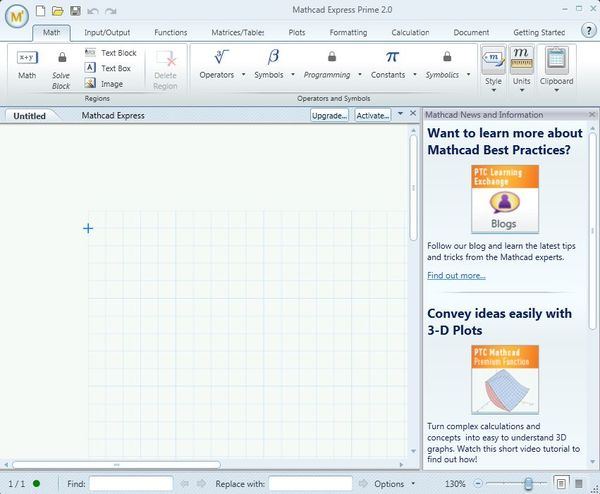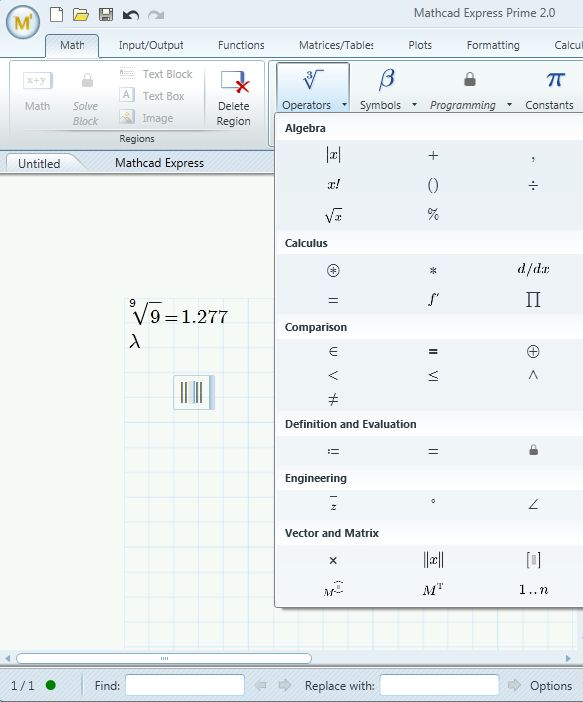Editor Ratings:
User Ratings:
[Total: 0 Average: 0]

Mathcad Express is a free mathematical computation software for Windows which you can use for various advanced mathematical calculations. You can probably notice from the fact that theres CAD in its name that this free mathematical calculation software is aimed more at engineers who need to perform various advanced calculations related to engineering problems. That’s the area where Mathcad Express is most useful, but other mathematicians can also use it.

Image down below shows us the interface of Mathcad Express. It basically consists of three parts. At the top we have the toolbar where all the important options for managing the math problems that you decide to setup can be seen. Mathematical problems that you’re working on can be seen down below. Problem editing area takes up the better part of the bottom left section of the interface, as you can see.Right sidebar has some introductory instructions which are gonna help you get familiar with this free mathematical calculation software. You can scroll through several instruction-type posts, but for the most part you’re gonna have to have at least some understanding of math to setup problems using this free software.

## Key features of Mathcad Express are:

• Free mathematical software for creating complex mathematical CAD models
• Allows you to open up Excel spreadsheets and continue editing their data
• Management of both symbolic and numeric mathematical calculations
• 3D plotting: allows you to plot your calculations in a 3D fashion
• Fast performance when doing calculations: uses 64bit architecture
• Works with all versions of Windows: starting with Windows XP

Similar software: SMath Studio, Euler Math Toolbox, Red Crab.

Not all of the features that Mathcad usually has are available in the Express version. To use the full functionality of this free CAD mathematical calculation software, you’re gonna have to upgrade to the professional version. To download the free version, you also have to setup an account on the Mathcad website.

## How to perform math calculations with Mathcad Express: free math calculation software

We’re not gonna go into advanced mathematical problems, because we’re not entirely sure how to set them up ourselves. It basically boils down to clicking on the Add Math button, which creates a new math problem box on the editing area down below.Just use the toolbar at the top if you’d like to insert special characters into your math problem. As soon as you’re done typing all the date, click Enter. As you do that, the problem should be solved. Like we said, for something more advanced, you’re gonna have to have math know-how, but Mathcad Express is more than capable of it. Solving problem, generating functions, 3D plotting, you name it, this free math calculation software can do it.

## Conclusion

Mathcad Express has a lot of interesting functions, which are gonna make your math calculations a lot more easier on you. It works fast and despite being a bit larger application, around 500MB, you don’t have to have too much of an advanced computer in order to run everything smoothly and without freezes. If you’re a CAD engineer who does a lot of math, try this math software and let us know how it goes in the comment section down below.